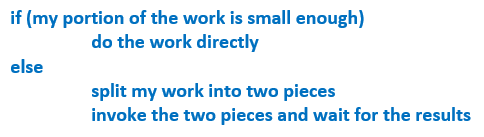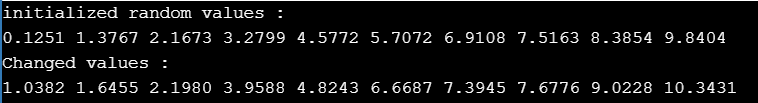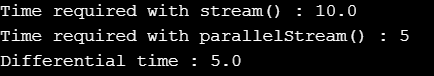# Parallel Programming in Java

## Parallel Programming in Java with Examples

In this article, I am going to discuss Parallel Programming in Java with Examples. Please read our previous article where we discussed Regular Expression in Java with Examples. At the end of this article, you will understand what is Parallel Programming and why need Parallel Programming as well as How to implement Parallel Programming in Java with Examples.

##### Why Parallel Programming?

With the advent of multicore CPUs in recent years, parallel programming is the way to take full advantage of the new processing workhorses. Parallel programming refers to the concurrent execution of processes due to the availability of multiple processing cores. This, in essence, leads to a tremendous boost in the performance and efficiency of the programs in contrast to linear single-core execution or even multithreading. It actually involves dividing a problem into subproblems, solving those problems simultaneously, and then combining the results of the solutions to the subproblems. Java SE provides the fork/join framework, which enables you to more easily implement parallel programming in your applications. However, with this framework, you want to specify how the issues are subdivided (partitioned). With aggregate operations, the Java runtime performs this partitioning and mixing of solutions for you.

##### What is Parallel Programming in Java?

Unlike multithreading, where each task is a discrete logical unit of a larger task, parallel programming tasks are independent and their execution order does not matter. The tasks are defined according to the function they perform or data used in processing; this is called functional parallelism or data parallelism, respectively. In functional parallelism, each processor works on its section of the problem whereas, in data parallelism, the processor works on its section of the data. Parallel programming is suitable for a larger problem base that does not fit into a single CPU architecture, or it may be the problem is so large that it cannot be solved in a reasonable estimate of time. As a result, when the tasks are distributed among processors, they can obtain the result relatively fast.

##### The Fork/Join Framework in Java

The Fork/Join Framework has been defined in the java.util.concurrent package. It consists of several classes and interfaces that support parallel programming. The notable difference between multithreading and parallel programming with this framework is: Here, the processing part is optimized to use multiple processors unlike multithreading, where the idle time of the single CPU is optimized on the basis of shared time. Using multithreading in a parallel execution environment is the added advantage of this framework. It is an implementation of the ExecutorService interface that helps you take advantage of multiple processors.

###### Syntax:And then, wrap this code in a ForkJoinTask subclass, typically using one of its abstract tasks: RecursiveAction and RecursiveTask.

##### Classes
1. RecursiveAction: It does not return any result; you can use it e.g. to initialize a big array with some custom values. Each subtask works alone on its own piece of that array. To create a RecursiveAction, you need to create your own class which extends from java.util.Concurrent.RecursuveAction (which is actually an abstract class) and implement its abstract method compute().
2. To call a RecursiveAction, you need to create a new instance of your RecursiveAction implementation and invoke it using ForkJoinPool.
3. RecursiveTask: It is appropriate when you need to return a result from your task, e.g. sorting a really huge array. The result of each subtask needs to be compared with each other. This task is a little bit harder to code.
4. ForkJoinTask: This is an abstract class that defines a task. Typically, a task is created with the help of the fork() method defined in this class. It is similar to a normal thread created with the Thread class but is lighter than it.
5. ForkJoinPool: It provides a common pool to manage the execution of ForkJoinTask tasks. It basically provides the entry point for submissions from non-ForkJoinTask clients, as well as management and monitoring operations.
##### Methods
1. Compute(): When you call compute() on the right Task actually you are doing a recursive call.
2. Fork(): The calling of the fork() method places our newly created PrimeRecursiveAction in the current thread’s task queue.
3. Join(): When you call join() on the left (previously forked) task, it should be one of the last steps after calling fork() and compute(). Calling join() means that “I can’t continue unless this (left) task is done.” But calling join() is not only about waiting. The task you call join() on can still be in the queue (not stolen). In this case, the thread calling join() will execute the joined task.
##### The Fork/Join Framework Strategy: divide-and-conquer strategy

This framework uses a divide-and-conquer strategy to implement parallel processing. It basically divides a task into smaller subtasks; then, each subtask is further divided into sub-subtasks. This process is applied recursively to each task until it is small enough to be handled sequentially. Suppose we are to increment the values of an array of N numbers. This is the task. Now, we can divide the array by two creating two subtasks. Divide each of them again into two more subtasks, and so on. In this way, we can apply a divide-and-conquer strategy recursively until the tasks are singled out into a unit problem. This unit problem is then executed in parallel by the multiple core processors available. In a non-parallel environment, we have to cycle through the entire array and do the processing in sequence. This is clearly an inefficient approach in view of parallel processing.

##### Example to implement Parallel Programming in Java by using Fork/Join Framework
```import java.util.concurrent.RecursiveAction;
import java.util.concurrent.ForkJoinPool;
import java.util.concurrent.TimeUnit;
public class ParallelismDemo1 extends RecursiveAction
{
final int THRESHOLD = 2;
double[] numbers;
int indexStart, indexLast;

ParallelismDemo1 (double[]n, int s, int l)
{
numbers = n;
indexStart = s;
indexLast = l;
}

protected void compute ()
{
if ((indexLast - indexStart) > THRESHOLD)
for (int i = indexStart; i < indexLast; i++)
numbers[i] = numbers[i] + Math.random ();
else
invokeAll (new ParallelismDemo1 (numbers,
indexStart,
(indexStart - indexLast) / 2),
new ParallelismDemo1 (numbers, (indexStart - indexLast) / 2,
indexLast));
}
public static void main (String[]args)
{
final int SIZE = 10;
ForkJoinPool pool = new ForkJoinPool ();
double na[] = new double[SIZE];
System.out.println ("initialized random values :");
for (int i = 0; i < na.length; i++)
{
na[i] = (double) i + Math.random ();
System.out.format ("%.4f ", na[i]);
}
System.out.println ();
ParallelismDemo1 task = new ParallelismDemo1 (na, 0, na.length);
System.out.println ("Changed values :");
for (int i = 0; i < 10; i++)
System.out.format ("%.4f ", na[i]);
System.out.println ();
}
}
```
###### Output##### Parallel Stream Execution in Java

We can execute streams in serial or in parallel. When a stream is executing in parallel, the Java runtime partitions the stream into multiple substreams. Aggregate operations iterate over and process these substreams in parallel then combine the results.

When you create a stream, it is always a serial stream unless otherwise specified. To create a parallel stream, invoke the operation Collection.parallelStream. Alternatively, invoke the operation BaseStream.parallel.

For example, the following statement calculates the average age of all male members in parallel:
double average = roster
.parallelStream()
.filter(p -> p.getGender() == Person.Sex.MALE)
.mapToInt(Person::getAge)
.average()
.getAsDouble();

##### Example to implement Parallel Stream in Java
```import java.util.ArrayList;
import java.util.List;

public class ParallelStreamDemo
{
public static void main (String[]args)
{
List < Integer > numList = new ArrayList < Integer > ();
for (int i = 0; i < 1000; i++)
{
}

// Processing sequentially
long startTime = System.currentTimeMillis ();
numList.stream ().forEach (i->processData (i));
long endTime = System.currentTimeMillis ();
double sequentialStreamTimetaken = (endTime - startTime) / 1000;
System.out.println ("Time required with stream() : " + sequentialStreamTimetaken);

// Parallel processing
startTime = System.currentTimeMillis ();
numList.parallelStream ().forEach (i->processData (i));
endTime = System.currentTimeMillis ();
long parallelStreamTimetaken = (endTime - startTime) / 1000;
System.out.println ("Time required with parallelStream() : " + parallelStreamTimetaken);
System.out.println ("Differential time : " +(sequentialStreamTimetaken - parallelStreamTimetaken));
}

private static void processData (int num)
{
try
{
} catch (InterruptedException e)
{
e.printStackTrace ();
}
}
}
```
###### OutputNote: Parallelism is not automatically faster than performing operations serially, although it can be if you have enough data and processor cores. While aggregate operations enable you to more easily implement parallelism, it’s still your responsibility to work out if your application is suitable for parallelism.

In the next article, I am going to discuss Reflection in Java with Examples. Here, in this article, I try to explain Parallel Programming in Java with Examples. I hope you enjoy this Parallel Programming in Java with Examples article.